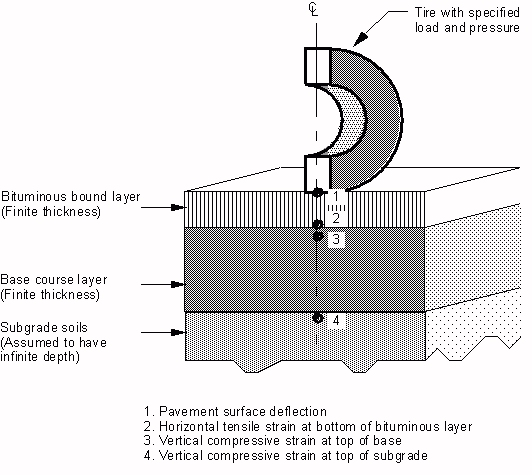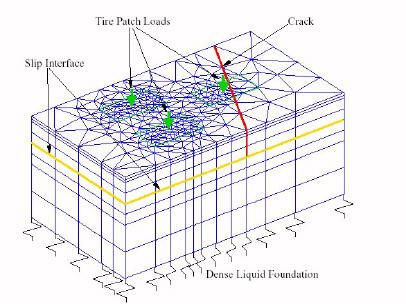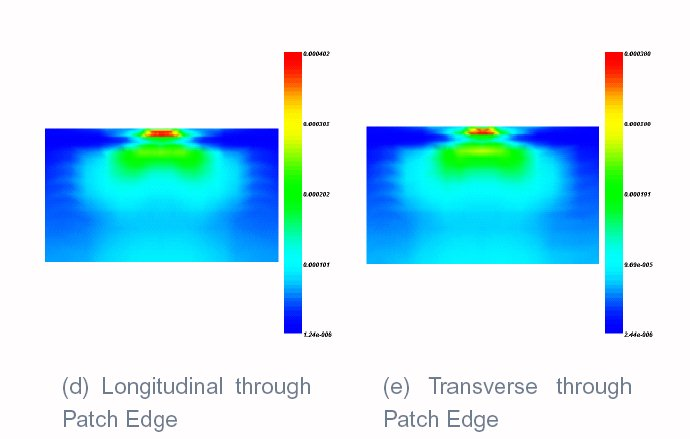# Flexible Pavement Mechanistic Models

Mechanistic models are used to mathematically model pavement physics.  There are a number of different types of models available today (e.g., dynamic, viscoelastic models) but this section will present two, the layered elastic model and the finite elements model (FEM), as examples of the types of models typically used.  Both of these models can easily be run on personal computers and only require data that can be realistically obtained.

## Layered Elastic Model

A layered elastic model can compute stresses, strains and deflections at any point in a pavement structure resulting from the application of a surface load.  Layered elastic models assume that each pavement structural layer is homogeneous, isotropic, and linearly elastic.  In other words, it is the same everywhere and will rebound to its original form once the load is removed.  The origin of layered elastic theory is credited to V.J. Boussinesq who published his classic work in 1885.  Today, Boussinesq influence charts are still widely used in soil mechanics and foundation design.  This section covers the basic assumptions, inputs and outputs from a typical layered elastic model.

### Assumptions

The layered elastic approach works with relatively simple mathematical models and thus, requires some basic assumptions.  These assumptions are:

• Pavement layers extend infinitely in the horizontal direction.
• The bottom layer (usually the subgrade) extends infinitely downward.
• Materials are not stressed beyond their elastic ranges.

### Inputs

A layered elastic model requires a minimum number of inputs to adequately characterize a pavement structure and its response to loading. These inputs are:

• Material properties of each layer
• Pavement layer thicknesses
• Magnitude. The total force (P) applied to the pavement surface
• Geometry. Usually specified as being a circle of a given radius (r or a), or the radius computed knowing the contact pressure of the load (p) and the magnitude of the load (P).  Although most actual loads more closely represent an ellipse, the effect of the differences in geometry become negligible at a very shallow depth in the pavement.
• Repetitions.  Multiple loads on a pavement surface can be accommodated by summing the effects of individual loads.  This can be done because we are assuming that the materials are not being stressed beyond their elastic ranges.

Figure 1 shows how these inputs relate to a layered elastic model of a pavement system.

#### Output

The outputs of a layered elastic model are the stresses, strains, and deflections in the pavement:

• Stress.  The intensity of internally distributed forces experienced within the pavement structure at various points.  Stress has units of force per unit area (N/m2, Pa or psi).
• Strain.  The unit displacement due to stress, usually expressed as a ratio of the change in dimension to the original dimension (mm/mm or in/in).  Since the strains in pavements are very small, they are normally expressed in terms of microstrain (10-6).
• Deflection.  The linear change in a dimension.  Deflection is expressed in units of length (mm or µm or inches or mils).

The use of a layered elastic analysis computer program will allow one to calculate the theoretical stresses, strains, and deflections anywhere in a pavement structure.  However, there are a few critical locations that are often used in pavement analysis (Table 1 and Figure 2).

Table 1. Critical Analysis Locations in a Pavement Structure

Location Response Reason for Use
Pavement Surface Deflection Used in imposing load restrictions during spring thaw and overlay design (for example)
Bottom of HMA layer Horizontal Tensile Strain Used to predict fatigue failure in the HMA
Top of Intermediate Layer (Base or Subbase) Vertical Compressive Strain Used to predict rutting failure in the base or subbase
Top of Subgrade Vertical Compressive Strain Used to predict rutting failure in the subgradeFigure 2. Critical analysis locations in a pavement structure.

## Finite Elements Model

The finite element method (FEM) is a numerical analysis technique for obtaining approximate solutions to a wide variety of engineering problems.  Although originally developed to study stresses in complex airframe structures, it has since been extended and applied to the broad field of continuum mechanics (Huebner et al., 2001).  In a continuum problem (e.g., one that involves a continuous surface or volume) the variables of interest generally possess infinitely many values because they are functions of each generic point in the continuum.  For example, the stress in a particular element of pavement cannot be solved with one simple equation because the functions that describe its stresses are particular to its specific location.  However, the finite element method can be used to divide a continuum (e.g., the pavement volume) into a number of small discrete volumes in order to obtain an approximate numerical solution for each individual volume rather than an exact closed-form solution for the whole pavement volume.  Fifty years ago the computations involved in doing this were incredibly tedious, but today computers can perform them quite readily.

In the FEM analysis of a flexible pavement, the region of interest (the pavement and subgrade) is discretized into a number of elements with the wheel loads are at the top of the region of interest (Figure 3).  The finite elements extend horizontally and vertically from the wheel to include all areas of interest within the influence of the wheel.Figure 3. EverFlex 3-D drawing (adapted from Wu, 2001). The drawing shows the discrete elements, wheel loads (tire patch loads), a modeled crack and a slip interface (where on layer can slip – move independently – from another).

### Assumptions

The FEM approach works with a more complex mathematical model than the layered elastic approach so it makes fewer assumptions.  Generally, FEM must assume some constraining values at the boundaries of the region of interest.  For instance, the computer program developed by Hongyu Wu and George Turkiyyah at the University of Washington (Wu, 2001), called EverFlex, uses a 6-noded foundation element to model the Winkler Foundation.  This program also uses free boundaries on the four sides of the flexible pavement model.  Additionally, the choice of element geometry (size and shape) as well as interpolation functions will influence overall model performance.

### Inputs

The typical finite elements method approach involves the following seven steps (Huebner et al., 2001):

1. Discretize the Continuum.  The region of interest is divided into small discrete shapes.
2. Select Interpolation Functions.  Nodes are assigned to each element and then a function is chosen to interpolate the variation of the variable over the discrete element.
3. Find the Element Properties.  Using the established finite element model (the elements and their interpolation functions) to determine matrix equations that express the properties of the individual elements.
4. Assemble the Element Properties to Obtain the System Equations.  Combine the matrix equations expressing the behavior of the elements and form the matrix equations expressing the behavior of the entire system.
5. Impose the Boundary Conditions.  Impose values for certain variables at key boundary positions (e.g., the bottom and sides of the chosen region of analysis).
6. Solve the System Equations.  The above process results in a set of simultaneous equations that can then be solved.
7. Make Additional Computations If Desired.  The unknowns are displacement components.  From these displacements element strains and stresses can be calculated.

### Outputs

The outputs of a FEM analysis are the same as for a layered elastic model:

• Stress.  The intensity of internally distributed forces experienced within the pavement structure at various points.  Stress has units of force per unit area (N/m2, Pa or psi).
• Strain.  The unit displacement due to stress, usually expressed as a ratio of the change in dimension to the original dimension (mm/mm or in/in).  Since the strains in pavements are very small, they are normally expressed in terms of microstrain (10-6).
• Deflection.  The linear change in a dimension.  Deflection is expressed in units of length (mm or µm or inches or mils).

In addition, the finite elements method allows for extremely powerful graphical displays of these values (Figures 4 through 7).Figure 4. 3-D Strain diagram.Figure 5. Surface strain diagram.Figure 6. Section view strain diagram.Figure 7. Sample load profiles. Screen Shot Thumbnails from EverFlex (Wu, 2001). Click on each thumbnail to see a larger version of the picture.

Footnotes    (↵ returns to text)
1. The Finite Element Method for Engineers, 4th Edition.  by John Wiley & Sons, Inc.  New York, NY.
2. Parallel Methods for Static and Dynamic Simulation of Flexible Pavement Systems.  Doctoral Dissertation.  University of Washington.  Seattle, WA.